## Monday, September 5, 2016

### An interesting Interview Question: Fibonacci Sequence

Write a function to calculate the nth Fibonacci Sequence is a common interview question and often the solution is something like

int Fib(int n)

{

if(n < 1) return 1;

return Fib(n-1) + Fib(n-2);

}

The next question is to ask for n=100, how many items will be on the stack. The answer is not 100 but actually horrible! It is closer to 2^100.

take the first call – we start a stack on Fib(99) and one on Fib(98). There is nothing to allow Fib(99) to borrow the result of Fib(98).  So one step is two stack items to recurse.  Each subsequent call changes one stack item into 2 items.   For example

• 2 –> call [Fib(1), Fib(0)]
• 3 –> calls [ Fib(2)->[Fib(1), Fib(0)], Fib(1) –> Fib(0) ]
• 4 –> calls [ Fib(3)->[[[ Fib(2)->[Fib(1), Fib(0)], Fib(1) –> Fib(0) ]], Fib(2)->[Fib(1), Fib(0)], Fib(1) –> Fib(0) ]

Missing this issue is very often seen with by-rote developers (who are excellent for some tasks).

A better solution is to cache the values as each one is computed – effectively creating a lookup table. You are trading stack space for memory space.

Placing constraints on memory and stack space may force the developer to do some actual thinking. A solution that conforms to this is shown below

private static long Fibonacci(int n) {
long a = 0L;
long b = 1L;
for (int i = 31; i >= 0; i—)  //31 is arbitrary, see below

{

long d = a * (b * 2 - a);
long e = a * a + b * b;
a = d;
b = e;
if ((((uint)n >> i) & 1) != 0) {
long c = a + b;
a = b;
b = c;
}
}
return a;
}

The output of the above shows what is happening  and suggests that the ”31”  taking the log base 2 of N can likely be done to improve efficiency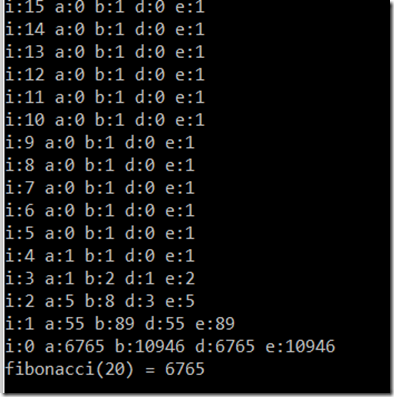for 32: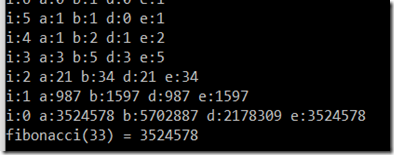for 65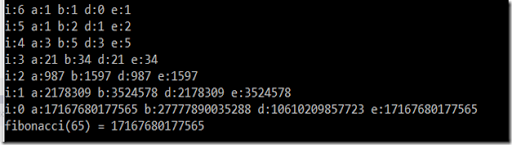for 129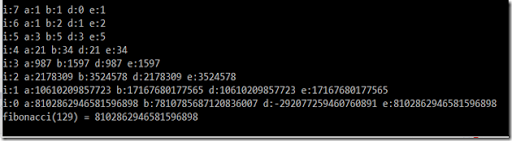What is the difference in performance for the naive vs the latter?

I actually did not wait until the naive solution finished… I aborted at 4 minutes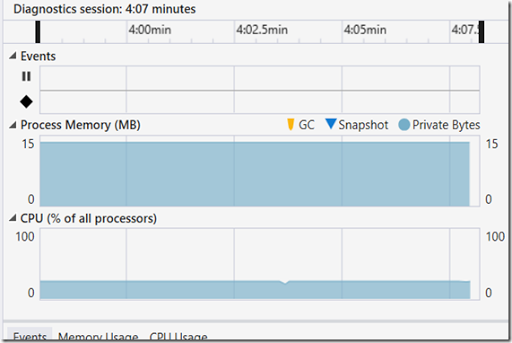The new improved version was 85 ms, over a 3000 fold improvement.

# Take Away

This question:

1. Identify if a person knows what recursion is and can code it.
2. Identify if he understands what the consequence of recursion is and how it will be executed(i.e. think about what the code does)
1. Most recursion questions are atomic (i.e. factorial) and not composite (recursion that is not simple)
3. Is able to do analysis of a simple mathematical issue and generate a performing solution.

#### 1 comment:

1.